# CS 5321 Numerical Optimization 07 352021 LargeScale Unconstrained

• Slides: 9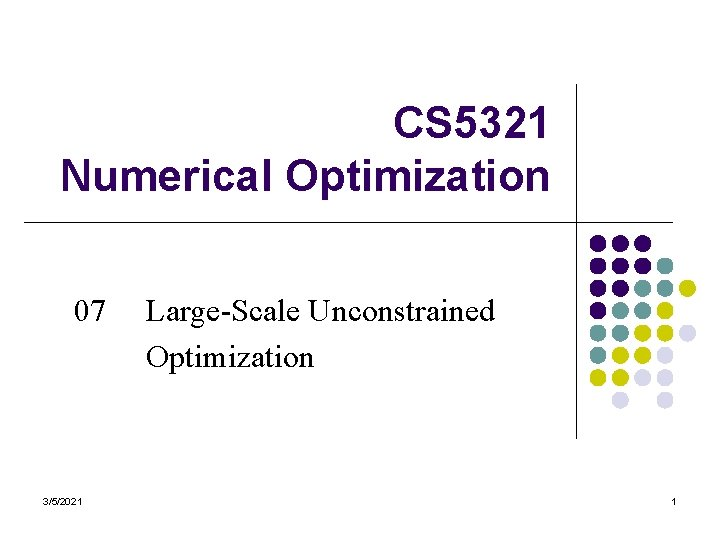CS 5321 Numerical Optimization 07 3/5/2021 Large-Scale Unconstrained Optimization 1Large-scaled optimizations l l The problem size n may be thousands to millions. Storage of Hessian is n 2. l l Even if it is sparse, its decompositions (LU, Cholesky…) and its approximations (BFGS, SR 1…) are not. Methods l Inexact Newton methods l l 3/5/2021 Line-search: Truncated Newton method Trust region: Steihaug’s algorithm Limited memory BFGS, Sparse quasi-Newton updates Algorithms for partially separable functions 2Inexact Newton method l Inexact Newton methods use iterative methods to solve the Newton’s direction p = −H− 1 g inexactly. l l l The exactness is measured by the residual rk=Hkpk+gk It stops when ||rk|| k||gk|| for 0< k <1. Convergence (Theorem 7. 1, 7. 2) If H is spd for x near x*, and x 0 is close enough to x*, the inexact Newton method converges to x*. If k 0, the convergence is superlinear. In addition, if H is Lipschitz continuous for x near x*, the convergence is quadratic. 3/5/2021 3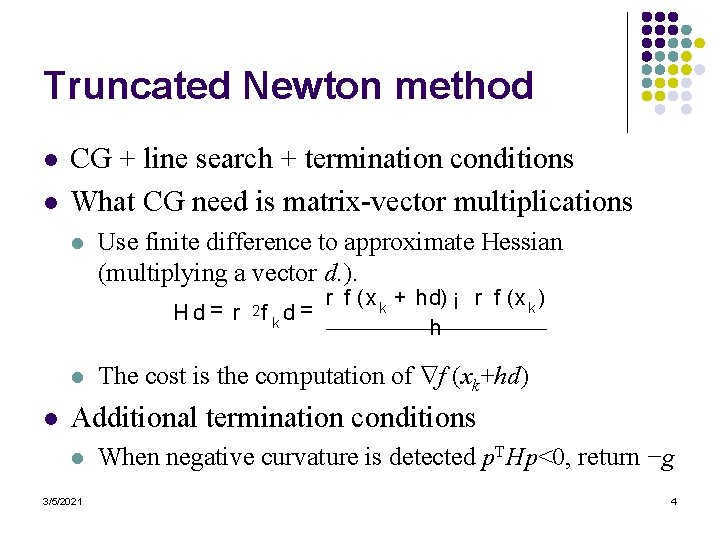Truncated Newton method l l CG + line search + termination conditions What CG need is matrix-vector multiplications l Use finite difference to approximate Hessian (multiplying a vector d. ). H d = r 2 f k d = l l r f (x k + hd) ¡ r f (x k ) h The cost is the computation of f (xk+hd) Additional termination conditions l When negative curvature is detected p. THp<0, return −g 3/5/2021 4Steihaug’s algorithm CG + trust-region + termination conditions l l Change terminations of CG: x z, p d. Index j Additional termination conditions l 1. 2. When negative curvature is detected p. THp<0, When the step size ||zj|| k, l l 3/5/2021 It can be shown that ||zj|| increases monotonically When stops abnormally, it returns zj+ dj, where minimizes mk(zj+ dj) subject to || zj+ dj ||= k. 5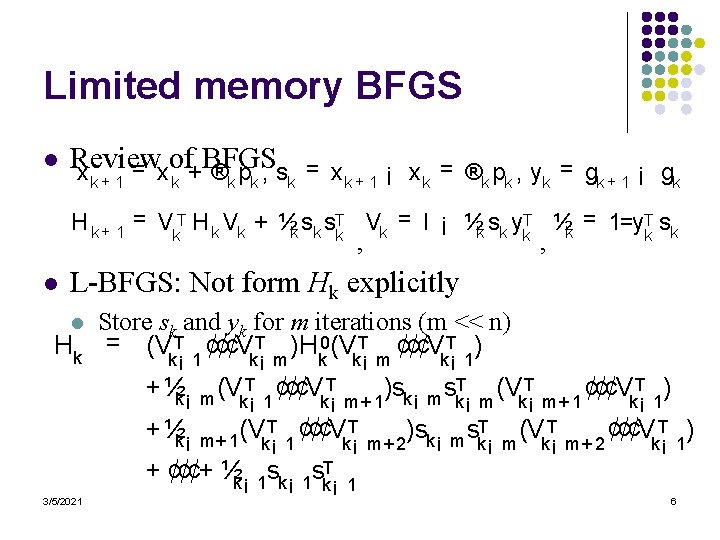Limited memory BFGS l Review of BFGS = x x + ®p , s k+ 1 k k = x k + 1 ¡ x k = ®k pk , yk = gk + 1 ¡ gk H k + 1 = Vk. T H k Vk + ½k sk sk. T Vk = I ¡ ½k sk yk. T ½k = 1=yk. T sk , l , L-BFGS: Not form Hk explicitly Store sk and yk for m iterations (m << n) Hk = (Vk¡T 1 ¢¢¢Vk¡T m )Hk 0(Vk¡T m ¢¢¢Vk¡T 1) l +½k¡ m (Vk¡T 1 ¢¢¢Vk¡T +½k¡ m+ 1 (Vk¡T 1 ¢¢¢Vk¡T + ¢¢¢+ ½k¡ 1 s. Tk¡ 3/5/2021 T T s )s (V m+ 1 k¡ m k¡ ¢¢¢V T ) m+ 1 k¡ 1 T T s )s (V m k¡ m+ 2 k¡ m k¡ ¢¢¢V T ) m+ 2 k¡ 1 1 6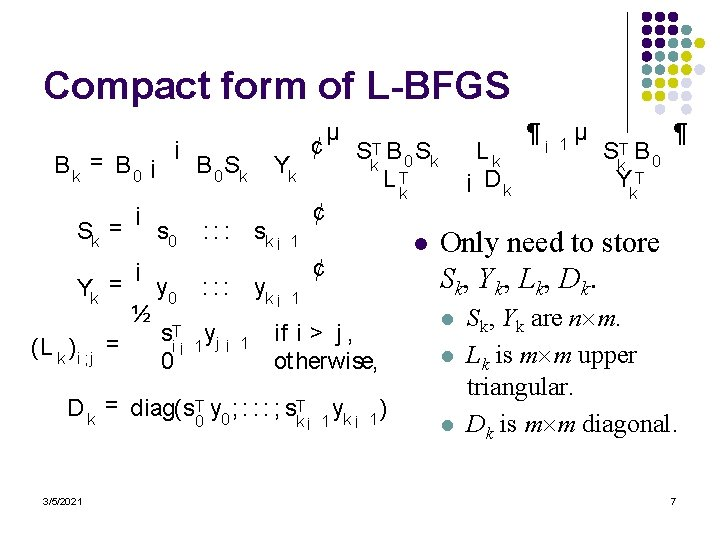Compact form of L-BFGS Bk = B 0 ¡ Sk = Yk = (L k ) i ; j = ¡ ¡ ½ ¡ s 0 y 0 B 0 Sk : : : sk ¡ : : : yk ¡ si. T¡ 1 yj ¡ 1 0 Yk ¢ µ ¢ Sk. T B 0 Sk L k. T 1 ¢ 1 if i > j , ot herwise, D k = diag(s 0 T y 0 ; : : : ; sk. T¡ 1 yk ¡ 1 ) 3/5/2021 l Lk ¡ Dk ¶¡ 1µ Sk. T B 0 Yk. T ¶ Only need to store Sk, Yk, Lk, Dk. l l l Sk, Yk are n m. Lk is m m upper triangular. Dk is m m diagonal. 7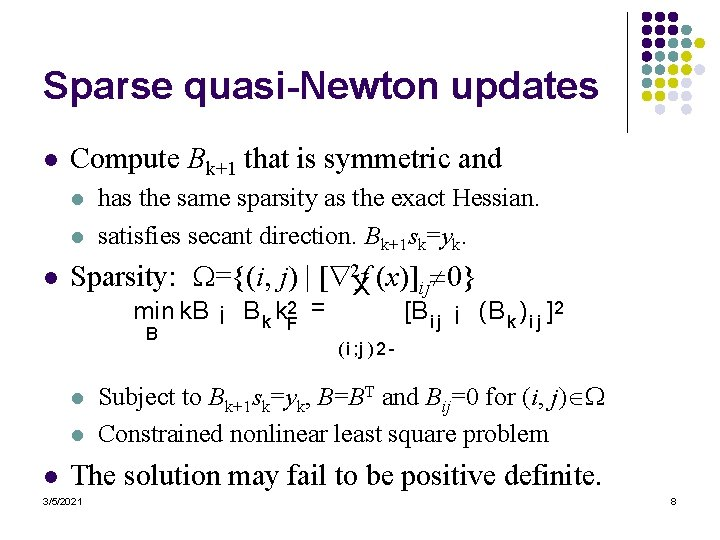Sparse quasi-Newton updates l Compute Bk+1 that is symmetric and l l l has the same sparsity as the exact Hessian. satisfies secant direction. Bk+1 sk=yk. Sparsity: ={(i, j) | [ 2 Xf (x)]ij 0} min k. B ¡ B k k 2 F = B l l l [B i j ¡ (B k ) i j ]2 ( i ; j ) 2 Subject to Bk+1 sk=yk, B=BT and Bij=0 for (i, j) Constrained nonlinear least square problem The solution may fail to be positive definite. 3/5/2021 8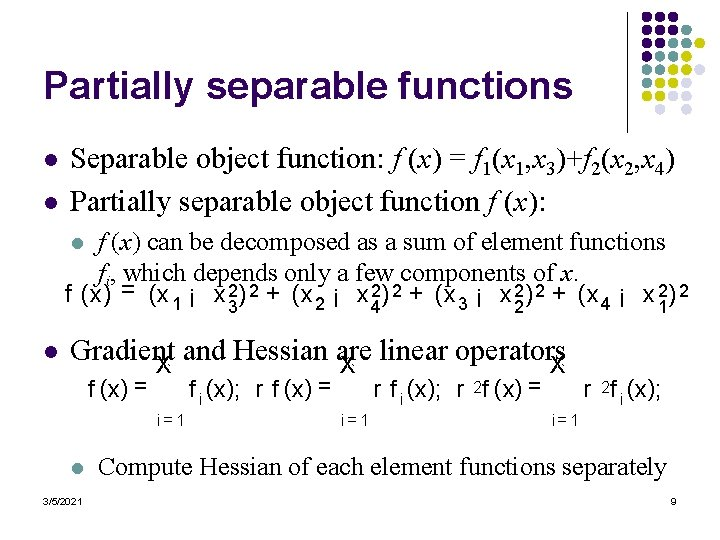Partially separable functions l l Separable object function: f (x) = f 1(x 1, x 3)+f 2(x 2, x 4) Partially separable object function f (x): f (x) can be decomposed as a sum of element functions fi, which depends only a few components of x. f (x) = (x 1 ¡ x 23 ) 2 + (x 2 ¡ x 24 ) 2 + (x 3 ¡ x 22 ) 2 + (x 4 ¡ x 21 ) 2 l l Gradient and Hessian are linear operators X X X f (x) = ` i=1 l 3/5/2021 f i (x); r f (x) = ` i=1 r f i (x); r 2 f (x) = ` r 2 f i (x); i=1 Compute Hessian of each element functions separately 9